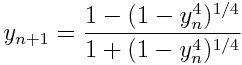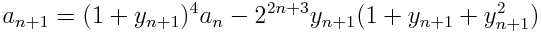Over a million developers have joined DZone.{{announcement.body}}
{{announcement.title}}

Algorithm of the Week: Algorithm Used for World Record Pi Calculations

DZone 's Guide to

Algorithm of the Week: Algorithm Used for World Record Pi Calculations

· Java Zone ·
Free Resource

Comment (0)

Save
{{ articles.views | formatCount}} Views

The following algorithm is based on work of Ramanujan and has been used in several world-record calculations of pi.

Initialize a0 = 6 – 4 √2 and y0 = √2 – 1. Then computeandThe terms an form a sequence of approximations to 1/π. The error in each approximation is given byThis says that the number of correct digits roughly quadruples after each step. To see this, note that the number of correct decimal places after the nth step is the negative of the logarithm base 10 of the error:[The error term goes to zero so quickly that you cannot (in ordinary precision) compute the error bound and then take logarithms; the exp(-2 π 4n) term will underflow to zero for n larger than 3. (See why here.) You have to take the log of the error term directly before evaluating numerically.]

The number of correct digits quadruples at each step because the leading term in the numerator above is 4n.

To give a few specifics, a1 is accurate to 9 digits, a2 is accurate to 41 digits, and a3 is accurate to 171 digits. After 10 steps, a10 is accurate to over 2.8 million digits.

The algorithm given here was state of the art as of 1989. It was used to set world records in 1986. I don’t know whether it has been used more recently. See more here.

According to this page, π has been calculated to 6.4 billion digits. You can beat this record if you can carry out 16 steps of the method described here. a16 would be accurate to over 11 billion digits.

Update: The algorithm used most recently for world record calculations for pi has been the Chudnovsky algorithm. As of October 2011, the record was over 10 trillion digits.

Topics:

Comment (0)

Save
{{ articles.views | formatCount}} Views

Published at DZone with permission of

Opinions expressed by DZone contributors are their own.